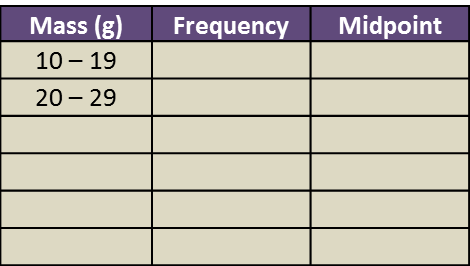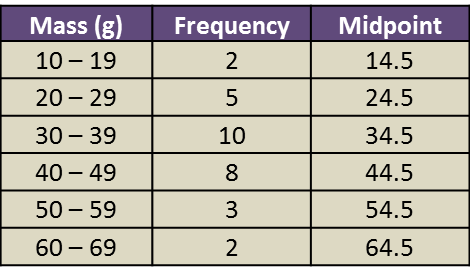6.7 SPM Practice (Long Questions)

Question 9 (12 marks):
The data in the Diagram shows the mass, in g, of 30 strawberries plucked by a tourist from a farm.Diagram

(a)
Based on the Diagram, complete Table 3 in the answer space.

(b)
Based on Table, calculate the estimated mean mass of a strawberry.

(c)
For this part of the question, use graph paper.
By using the scale of 2 cm to 10 g on the horizontal axis and 2 cm to 1 strawberry on the vertical axis, draw a histogram for the data.

(d)
Based on the histogram drawn in 14(c), state the number of strawberries with the mass of more than 50 g.Solution:
(a)(b)

(c)(d)
Number of strawberries with the mass more than 50 g
= 3 + 2
= 5